## RF Designer - Components (Ideal Transmission Line Elements)

Full listing and description of components that can be placed in an RF Designer schematic.
rftools
Posts: 13
Joined: Thu May 22, 2014 12:36 pm

### RF Designer - Components (Ideal Transmission Line Elements)

Ideal Transmission Line Elements

TLIN - Ideal Transmission Line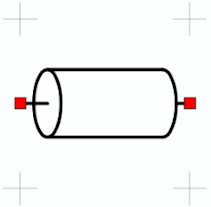Name Description Units Default Value Z Impedance Resistance 50 ohms EL Electrical length Degrees 90º F0 Frequency where EL is specified Frequency 1000 MHz

Model for an ideal and lossless transmission line. Electrical length (EL) is specified in degrees or radians at frequency F0.

TLOC - Ideal Open Circuited Transmission Line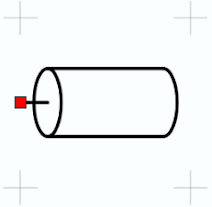Name Description Units Default Value Z Impedance Resistance 50 ohms EL Electrical length Degrees 45º F0 Frequency where EL is specified Frequency 1000 MHz

Model for an ideal and lossless open circuited transmission line. Electrical length (EL) is specified in degrees or radians at frequency F0.

TLSC - Ideal Short Circuited Transmission Line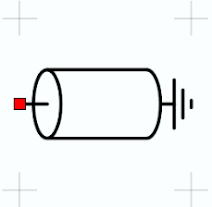Name Description Units Default Value Z Impedance Resistance 50 ohms EL Electrical length Degrees 45º F0 Frequency where EL is specified Frequency 1000 MHz

Model for an ideal and lossless short circuited transmission line. Electrical length (EL) is specified in degrees or radians at frequency F0.

TLIN4 - Ideal 4 Node Transmission Line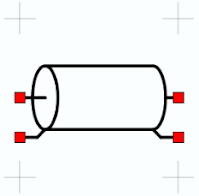Name Description Units Default Value Z Impedance Resistance 50 ohms EL Electrical length Degrees 90º F0 Frequency where EL is specified Frequency 1000 MHz

Model for an ideal and lossless transmission line with the ground terminals left open and available as ports in the schematic. Electrical length (EL) is specified in degrees or radians at frequency F0.

CLIN - Ideal Coupled Transmission Line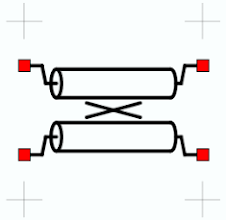Name Description Units Default Value Zoe Even-mode impedance Resistance 69 ohms Zoo Odd-mode impedance Resistance 36 ohms EL Electrical length Degrees 90º F0 Frequency where EL is specified Frequency 1000 MHz

Model for a pair of lossless symmetric coupled transmission lines. Electrical length is specified in degrees or radians at frequency F0. Coupling is determined by the even and odd mode impedances (Zoe and Zoo).
Given a desired coupling C in decibels, Zoe = Zo * sqrt((1 + k) / (1 - k)) where k = power(10.0, -C/20.0). Zoo can the be found by solving Zoo = Zo * sqrt((1 - k) / (1 + k))

Example: Find Zoe and Zoo for a 10 dB coupler in a 50 ohm system.
First calculate k as k = power(10.0, -10/20) = 0.316
Zoe is found as Zoe = Zo * sqrt((1 + .316) / (1 - .316)) = 69.4 ohms
Zoo is found as Zoo = Zo * sqrt((1 - .316) / (1 + .316)) = 36 ohms